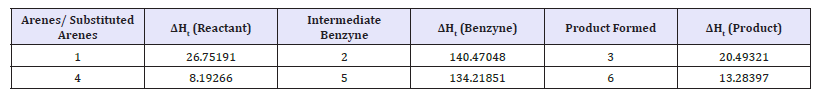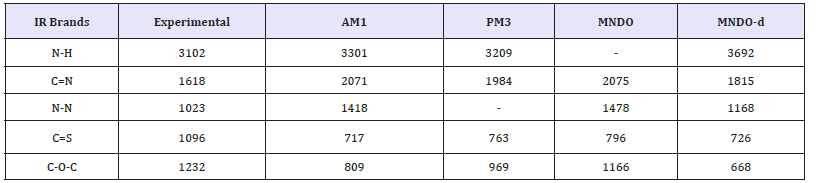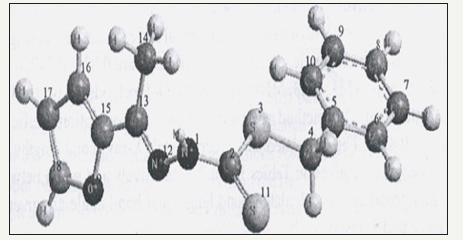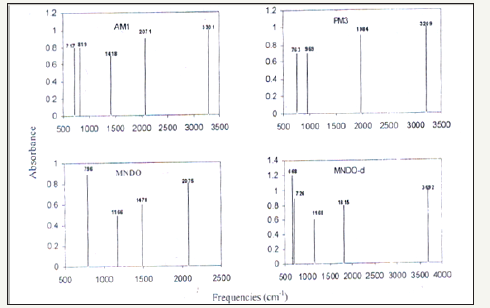• Submissions# Introduction to Computational Methods and Their Applications in Chemistry

Kishor Arora*

Department of Chemistry, Govt PG College (Autonomous), India

*Corresponding author:Kishor Arora, Department of Chemistry, Govt PG College (Autonomous), Datia (MP) 475 661, India

Submission: February 01, 2018;Published: May 30, 2018Volume2 Issue2
May 2018

#### Abstract

It is a demand in the modern era of chemical sciences to develop and design new and useful compounds for the service of mankind without the use of methods which are traditional one. Workers were trying to develop and synthesizing series of new compounds since past in the laboratories using traditional synthetic methods and they used to check their properties thereafter. This process is time consuming and this lead to synthesis of a large number of compounds. Some recent trends have been emerged and developed in the field of designing of compounds which may include microwave synthesis, computer aided designing etc. Out of these methods this present talk/presentation will focus on computer aided designing i.e. studying and designing of compounds of on the PC first without trying a lot of synthesis in the lab. These computational methods are less time consuming, involve less chances of error, helpful in controlling and causing less pollution and may prove to be helpful in designing compounds with desired properties. This paper/lecture includes introduction to the computational methods, a little theory behind these methods, their applications and use of software/s particularly use of software/s of semi-empirical methods in QSAR studies.

### Methods in science

Science is defined as the study of how nature behaves. Modern scientists agree that there are four methods in science:

a. Observational science

b. Experimental science

c. Theoretical science

d. Computational science

Let us focused on only one that is computational science.

### Computational science

a. Computational science is the application of computer science and mathematical techniques to the solution of large and complex problems.

b. Computational science allows scientists to do things that were previously too difficult to do due to the complexity of the mathematics, the large number of calculations involved, or a combination of the two.

c. Computational science allows scientists to build models that allow predictions of what might happen in the lab (Figure 1).

Figure 1:Computational science.### Molecular modelers and molecular modeling

Chemists are increasingly identifying themselves as molecular modelers and/or computational chemists. There are roughly two types of computational chemists: those who apply the techniques and tools of computational chemistry to solve interesting problems, and those who work to improve the techniques and tools [1-3]. Molecular modeling is rapidly becoming one of the important tools to be learned, molecular modeling allows the user to determine three fundamental items of interest of a molecule or system of molecules:

a. The structure, or geometry of the molecule

b. The property or properties of a molecule or system of molecules the activity, or reactivity, of a molecule or system of molecules.

### Fundamental approximations behind these techniques

a. The Born-Oppenheimer approximation: states that we ignore the motion of nuclei in molecules

b. The Hartree-Fock (HF) approximation: states that we can simplify our calculations by aggregating, or combining, the motion of electrons

c. The Linear Combination of Atomic Orbitals (LCAO): states that we can construct molecular orbitals by a relatively straightforward addition of calculated atomic orbitals. d. Most molecular modeling tools look to solve the Schrodinger Equation, In its most well known format, the Schrodinger equation is represented by:

HΨ = EΨ

Basically modeling is a technique of solving this equation within these three approximations as represented by the (Figure 2).

Figure 2:Basic model technique.### Some software/methods in use for this purpose

Figure 3:A comparative study of the packages/methods used/employed for modeling.There are three types of methods in use based on the consideration of electrons and formation of Hamiltonian to solve the Schrodinger equation set up for the molecular system [4-7]. These are viz. semi-empirical, ab-initio and density functional methods. Some common software which is in use now-a days are:

a. MOPAC

b. HYPERCHEM

c. GAMESS

d. Gaussian etc.

These are used with different basis sets which are chosen differently for different molecular system depending upon requirements.

A comparative study of the packages/methods used/employed for modeling is represented by the (Figure 3).

### Applications of semi empirical software which we have explored by our research group

Study of energy barriers for the reactions: Energy barriers for the reactions can be studied using such quantum chemical software’s. One such study confirms the benzyne intermediate formation in the conversion of halo benzene to amino benzene (Table 1). The proposed Mechanism is :

Table 1:AM1 computed heat of formation (Kcal mol-1) of the reactant haloarenes/substituted haloarenes, intermediate benzynes and the product amino arenes.Figure :Figure 4:Reaction profileTheoretical studies of electronic and geometric structures of the compounds: Theoretical studies of electronic and geometric structures of compounds can be done successfully using these packages. Some of such studies are carried out on organic compounds which are used as ligands in the formations of metal complexes. Such studies were carried out on Schiff base ligands some of them are shown below

Figure :Such studies shown that electron densities in Schiff bases are ample and maximum on azomethinic nitrogen. This fact supports that these Schiff bases can coordinate to central metal atom through azomethinic nitrogen [8-12].

Table 2:Experimental and calculated fundamental vibrational frequencies of S-Benzyl 1-b-M-(2-furyl methyl ketone) dithiocarbazate Schiff base of s-benyl dithiocarbazate by AM1, PM3, MANDO and MNDO-d.Figure 5:S-Benzyl 1-b-M-(2-furyl methyl ketone) dithio carbazate.### Computer simulation of IR spectra using these packages:

Normal modes analysis of some of the compounds can also be performed using specific keywords with the help of these computer packages. Computer aided spectral simulation of IR spectra of compounds can be done on the basis of normal mode analysis (Table 2). One such study for S-Benzyl 1-b-M-(2-furyl methyl ketone) dithio carbazate schiff base has been carried out with AM1, PM3 MNDO and MNDO-d methods. These computer simulated IR spectra are shown in (Figure 5,6).

Figure 6:Spectrum.QSAR Studies using these packages: QSAR studies include establishment of correlation equations, relationships between variations in the values of various molecular properties with their biological activities for the series of compounds. This is a common technique now-a –days used in designing of a drug. In most general form QSAR equation may be written as a linear equation: (Biological activity) = C+ m1p1+m2p2+……

In the equation shown above m’s are coefficients for the parameters or properties and “c” is a constant

It is clear from the equation that biological activity (or log of it) is function of properties/parameters.

QSAR technique: Various types of descriptors used in QSAR technique

1. Constitutional descriptors

2. Molecular weight

3. Counts of atoms and bonds

4. Counts of rings etc.

5. Topological descriptors

6. Connectivity indices

Now-a-days most of the workers are using software computed descriptors in QSAR techniques. These are in wide use. This computational Chemistry software provides structural properties/parameters which may be used to generate/establish QSAR equations by correlating biological activity of series of compounds under study.

Molecular modeling is rapidly becoming one of the important tools, along with basic lab skills and the use of specialized chemistry tools such as infrared spectrophotometers and spectroscopy instruments. Keeping this in mind, this presentation includes the QSAR studies of simple organic compounds which are carried out by our research group [13-17].

Computational details: Intel based Pentium core-2 Duo machine with configuration Intel (R) core TM 2 Duo CPU, T5450@1.66 GHZ, 2 GB RAM , 250GB HDD was used to run all the calculations. Semi-empirical AM1 (Austin modulated 1), and PM3 (Parameterized3) quantum chemical calculations were carried out by the computer software HYPERCHEM 8.0 version and calculated parameters such as normal modes frequencies of vibration.

#### Results and Discussion

Studies of Twelve pyrazole derivatives have been carried out for their antibacterial activity against S. aureus. All the compounds were screened for antibacterial activity at 200μg/ml concentration.

The analytical studies related to these compounds are reported in (Table 3).

Table 3:The analytical studies related to these compoundsThe best QSAR equations are given below

a. S. aureus/AM1

p ( M I C ) = - 0 . 0 0 0 1 3 ( S A A ) - 0 . 0 0 1 3 1 ( VO L ) + 0 . 0 1 7 4 0 6 ( HE)+0.461515

N=12, SD=0.071211, R=0.79799, F=4.675247

a. S. aureus/PM3

p(MIC)=-0.00716(HE)+1.16E-05(TE)+0.001534(HF)-0.22079 N=12, SD=0.058623, R=0.868243, F=8.166643

For the same series of Compounds the best QSAR equations, For the pathogen C. albicans correlation graphs between computed and observed activities are drawn on the basis of equation

b. C. albicans/AM1

p(MIC)=-0.0001261(SAA)-0.0013068(VOL)+0.01740588( HE)+0.46151539

N=12, SD=0.07121068, R=0.79799037, F=4.675247 b. C. albicans/

p(MIC)=-0.00056(SAA)+9.7E-06(TE)+0.001248(HF)-0.03723

N=12, SD=0.057574, R=0.873258, F=8.565155

Where symbols used in the above equations are SAA (surface area approx.); VOL (volume); HE (hydration energy); TE (total energy) and HF9 heat of formation) as computed using semiempirical quantum chemical software. From the discussion mentioned above it is clear those statistical tests and computed properties viz. SD(standard deviation), R(correlation coefficient), F-test along with regression analysis helps in selection of suitable QSAR equation and prediction of appropriate mathematical model [18-21].

#### Conclusion

QSAR studies pertaining to antifungal activities of the compounds (A1-A12) under study against the pathogen. This may be concluded form the studies that some of the compounds showed significant antifungal activity against a pathogen and other against other pathogen with related computed properties. This discussion also indicates that Quantitative Structure Activity Relationship equation in the light of statistical calculations or interpretations. In conclusion this may be concluded that statistics has a vital and significant role in QSAR studies and in predicting correct mathematical model and such type of studies may also be carried out using software, viz. ab-initio or semi-empirical, based calculations.

We at Crimson Publishing are a group of people with a combined passion for science and research, who wants to bring to the world a unified platform where all scientific know-how is available read more...